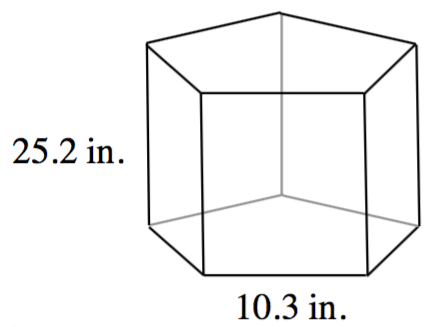### Home > CC1MN > Chapter 11 > Lesson 11.2.1 > Problem11-37

11-37.

The area of the base of the prism at right is $96.33$ square inches.

1. Find the surface area of the prism if the base is a regular pentagon.

The surface area is the sum of the areas of each individual face.

The top and bottom face both have an area of $96.33$ square inches.

There are five side faces, each with an area of
($25.2$ inches)($10.3$ inches), or $259.56$ square inches.

2. Find the volume of the prism.

Volume = (area of base)(height)

$\text{Volume}=(96.33\text{ in.}^2)(25.2\text{ in.})$

$\text{Volume }=2427.516\text{ in.}$

3. If another prism has the same volume as this prism, but its base area is $30$ square inches, what is its height?

Rearrange the equation used in part (b) to solve for height.